# Mathematics - Linear Algebra Method for solving Differential Equations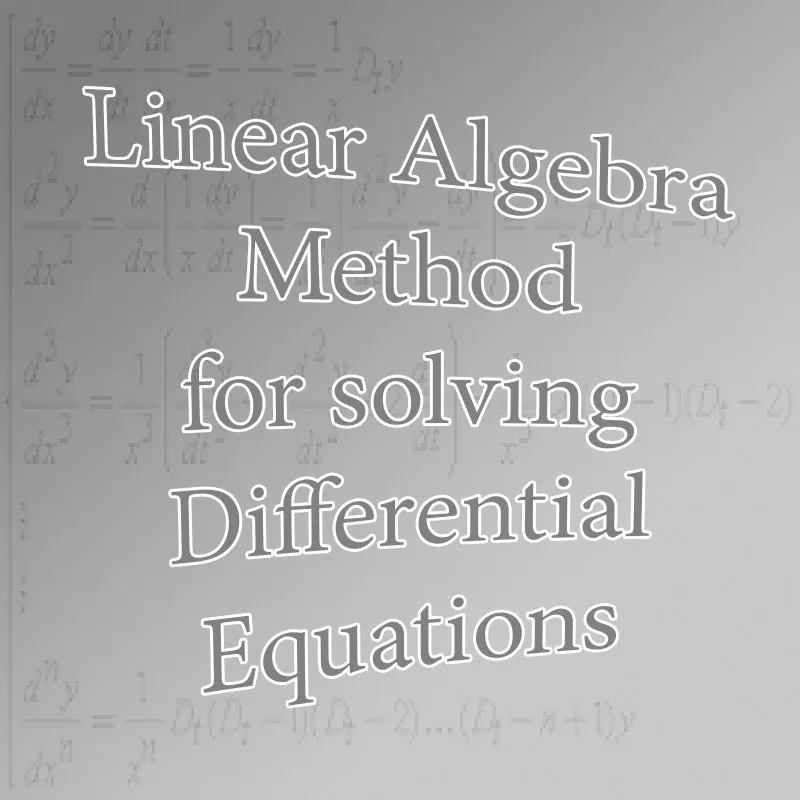## Introduction

Hello it's a me again drifter1! Today we get back to Mathematics to talk about Differential Equations again. In my Differential Equations series I covered many cases and solutions of ODE's, but there are still many more that are yet to be discovered and many more to talk about in general.

One thing that I promised to talk about is how we combine Linear Algebra (matrices) and Differential Equations to find solutions for linear ODE's that are of a much bigger degree then 1, 2, 3 that we where used to till now.

Using the Laplace Method we where able to solve any ODE, but the solutions came a little late! In the examples on specific known types you could clearly see that it took us much more time to solve using this Method then the simpler methods for the specific types we applied Laplace to!

So, what now? Well, for n-degree Linear ODE's we can use another method that takes us back to Linear Algebra. That's why I suggest you to go and read my previous posts about Linear Algebra that you can find in my recap here before getting into this post! Off course you should also check out my other posts about Differential Equations if you want to know more about ODE's in general. Links to the previous parts are in the end of this post!

All the equations in this post will be rendered using quicklatex.com

So, without further do, let's get started!

## N-order Linear ODE's

As already told in the Introduction we use Linear Algebra to solve N-order Linear Ordinary Differential Equations (ODE's).

A linear ODE has the following properties:

• All coefficients are functions of x (and not y)
• y and it's derivatives only come with power 1 (linearity).

So, such a ODE is of the form: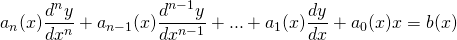Suppose that we also have the following startings conditions: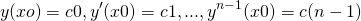Of course ai(x) and b(x) are functions of x with an(x) != 0 and ci being real numbers.

## Getting a linear system

The ODE of before can be rewritten to a new form by setting:That way we now have a ODE of this new form:Let's now define n new variables (equal to the degree n of our starting ODE):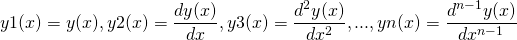This introduction of new variables gives us the opportunity of defining a linear system of (n-1) differential equations. These system looks like this:Let's not include yn yet.

The last of those equations let's us rewrite the derivative of yn like this: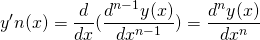And combining this with our first ODE we now get:So, our final linear system is:where y1(x), y2(x), ..., yn(x) are our unknown functions.

## Solving the linear system

So, we got the system what now?

Well, let's get into how we solve such a system...

Suppose the following linear system of 1st order ODE's:It's like the previous form, but more generalized!

The same way as we solved "normal" linear systems using: AX = b we this time define martices to solve Y' = AY + R.

A is the system matrix and is a nxn matrix of the form:Y is a column-matrix and contains the unknown functions yi(x). Y' is of course the column-matrix of the derivatives of yi(x). R on the other hand contains the non-homogeneous orders ri(x).

Those 3 look like this:And so the system we started with is now written with the matrix form:

Y' = AY + R

Of course the system is homogeneous if R = 0.

If Y1 and Y2 are two solutions of the homogeneous system then every linear combination c1Y1 + c2Y2 is of course also a solution of the homogeneous system (the same way as it did in "normal" equations!)

So, the solutions of a homogeneous differential equation of n-order will of course make up a n-dimensional vector space.

## Conversion Example

Write the following linear system in matrix form: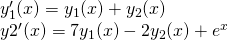The "special" matrices that represent the system are: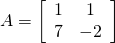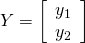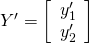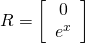And so the system is written like that: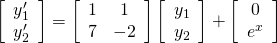## Theorems/Statements

1)

The generic solution Y of a non-homogeneous system Y' = AY + R is the sum of the general solution Y0 of the corresponding homogeneous system Y' = AY and the  partial solution Yp of the non-homogeneous one.

That way the generic solution is written:

Y = Y0 + Yp

This also applied in "normal" systems.

2)

If aij(x) and rj(x) are continuous in a range I then the linear system of differential equations has one solution Y(x) that fulfills the equation:At some point x0 in R that is defined in the whole range I.

## Wronsky Determinant

From this last statement we get into Wronsky determinants (that we also talked about in Linear Algebra).

Every n-pair of solutions y1(x), y2(x), ..., yn(x) can be written as a column-matrix.

We suppose that we have n-solutions ui of the form:Using all those as columns we end up wtith the matrix U that contains all the solutions of our system. This matrix is called the solution matrix:You might remember from Linear Algebra that a n-pair of functions g1(x), g2(x), ... gn(x) is linear independent if every linear combination c1g1 + c2g2 + ... + cngn = 0 leads us to the conclusion c1 = c2 = ... = cn =0 and nothing more! Else some pair of gi, gj and so the whole n-pair is linear dependent.

So, the previous solution matrix is fundamental only if the Wronsky Determinant is non-zero.

Which means that:## Linear system of 1st-order ODE's with constant coefficients

For the sake of simplicity let's just get into how we solve simple ones.

### Method

Suppose we have a homogeneous system Y' = AY.

Abel-Liouville's theorem tells us that: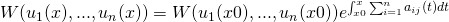For every x, xo in I (the range that we talked about earlier).

Because the aij coefficients are real numbers we can get the matrix of our system A in a form that is familiar to us from "normal" systems.

We know that the solution of a ODE dy/dx is ay with a being a constant and so of the form:
This points us to the conclusion that the solution of a homogeneous system dY/dx will also be of the form AY with:with l = λ being some nmber and a constant vector u of R^n.

If we think like that then:And because of the form of our system we have:Because of those last two equations we get to the conclusion that:From Linear Algebra we of course know that non-zero vectors u for which Au = λu, for some nxn matrix A and number λ are the Eigenvectors of the matrix A and λ is of course the Eigenvalue!

You can read about Eigenvalues/vectors here. (just so that you can get it faster!)

By guessing that the "form" stays the same we end up with the conclusion that the form is really the same and that dY/dx = AY with Y(x) = e^(λx) u with u being a  eigenvector of A for a eigenvalue λ.

So, finally the solutions of the homogeneous system dY/dx = AY can also be found by finding the eigenvalues and eigenvectors of the system matrix A.

### Example

Suppose the linear system: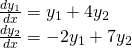Let's find the solutions of the system...

It can be written in the matrix form Y' = AY

So, the system matrix A is: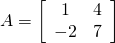And the matrices Y and Y' = dY/dx are:That way the system is written like that: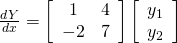The eigenvalues of A are the solutions of it's characteristic polynomial and so of: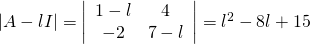which has the solutions λ1 = 3 and λ2 = 5.

Let's find the eigenvectors that correspond to each eigenvalue.

The eigenvectors x = [x1 x2] that correspond to λ1 = 3 can be found by solving the system:

AX = 3X <=> (A - 3I)X = 0 <=> -2x1 + 4x2 = 0 => x1 = 2x2.

So, the eigenvectors are of the form [x1 x2] = x2 [2 1].

Which means that a base of the eigenspace is the eigenvector u1 = [2 1]

In the same way, we can end up with the base-eigenvector u2 = [1 1] that corresponds to λ2 = 5...

So, two solutions of the system are: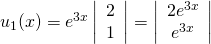And the generic solution of the system is: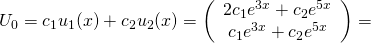If we also have the starting conditions: y1(0) = 1 and y2(0) = 2 then we can calculate the constant c1, c2.

That way:And so the only solution of our system would be: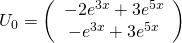Note that u1, u2 are linear independent and so U0 is a fundamental space of solutions!

This method can also be generalized to Complex numbers!

## Stuff related

Systems of 1st-order linear ODE's Notes

Matrix form conversion examples

E-book with ODE examples

## Previous posts of the series:

Introduction -> Definition and Applications

First-order part(1) ->  Separable, homogeneous and exact 1st-order ODE's

First-order part(2) -> Linear, Bernoulli and Riccati first-order ODE's

First-order exercises -> Exercises for all the 1st-order ODE types

Second-order linear with const coeffs -> Constant coefficient linear 2nd-order ODE's

Special second-order forms -> Linear Euler ODE, Wronsky and Lagrange (Canonical) methods

Second-order exercises -> Exercises for 2nd-order ODE's

Laplace method -> Laplace method for solving ODE's and systems of ODE's

Laplace method exercises -> Simple Examples for the Laplace method

And this is actually it for today and I hope that you enjoyed it!
Next time in Mathematics we will either get into a new branch that will become a series or talk about Trigonometry, the same way as I did for Complex numbers!

See ya!

H2
H3
H4
3 columns
2 columns
1 column
Join the conversation now